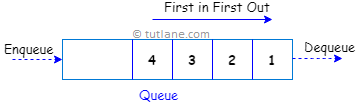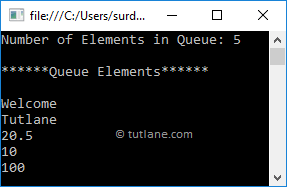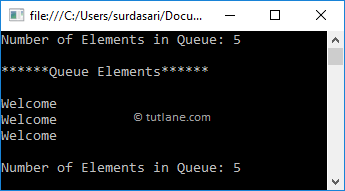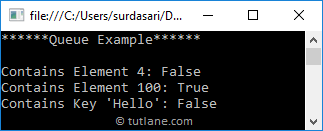# C# Queue with Examples

In c#, Queue is useful for representing a collection of objects that stores elements in FIFO (First In, First Out) style, i.e., the element that added first will come out first. In a queue, elements are inserted from one end and removed from another end.

Generally, queues are useful when you want to access elements from the collection in same order that is stored, and you can store multiple null and duplicate values in a queue based on our requirements.

Using Enqueue() and Dequeue() methods, we can add or delete an element from the queue. Here, the Enqueue() method is useful to add elements at the end of the queue, and the Dequeue() method is useful to remove elements starting from the queue.

Following is the pictorial representation of the queue process flow in the c# programming language.## C# Queue Declaration

Generally, c# will support both generic and non-generic types of queues. Here, we will learn about non-generic queue collections by using the System.Collections namespace.

As discussed, the collection is a class. To define a queue, you need to declare an instance of the queue class before performing any operations like add, delete, etc. like as shown below.

Queue que = new Queue();

If you observe the above queue declaration, we created a new queue (que) with an instance of a queue class without specifying any size.

## C# Queue Properties

The following are some of the commonly used properties of a queue in the c# programming language.

PropertyDescription
Count It will return the total number of elements in the queue
IsSynchronized It is used to get a value to indicate whether access to the queue is synchronized (thread-safe) or not.

## C# Queue Methods

The following are some of the commonly used queue methods to perform operations like add, delete, etc., on elements of a queue in the c# programming language.

MethodDescription
Enqueue It is used to add elements at the end of the queue.
Dequeue It will remove and returns an item from the start of a queue.
Clear It will remove all the elements from the queue.
Clone It will create a shallow copy of the queue.
Contains It is used to determine whether an element exists in a queue or not.
Peek It is used to get the first element from the queue.
TrimToSize It is used to set the capacity of a queue to an actual number of elements in the queue.

## C# Queue Example

Here, we will use a non-generic type of queue to add elements of different data types to the queue using Enqueue() method. Following is the example of adding and accessing elements of the queue in c#.

using System;
using System.Collections;

namespace Tutlane
{
class Program
{
static void Main(string[] args)
{
// Create and initialize a queue
Queue que = new Queue();
que.Enqueue("Welcome");
que.Enqueue("Tutlane");
que.Enqueue(20.5f);
que.Enqueue(10);
que.Enqueue(100);
Console.WriteLine("******Queue Example******");
Console.WriteLine("Number of Elements in Queue: {0}", que.Count);
Console.WriteLine("******Queue Elements******");
// Access Queue Elements
foreach (var item in que)
{
Console.WriteLine(item);
}
}
}
}

If you observe the above example, we created a new queue (que). We added different data type elements to the queue (que) using Enqueue() method, and we used a foreach loop to iterate through a queue to get elements from it.

When you execute the above c# program, you will get the result below.If you observe the above result, we got elements from the queue in the same order, which we added first.

## C# Dequeue() Method to Access Queue Elements

As discussed, the queue Dequeue() method will always remove and return the first element of the queue. Following is the example of accessing queue elements using the Dequeue() method in c#.

using System;
using System.Collections;

namespace Tutlane
{
class Program
{
static void Main(string[] args)
{
// Create and initialize a queue
Queue que = new Queue();
que.Enqueue("Welcome");
que.Enqueue("Tutlane");
que.Enqueue(20.5f);
que.Enqueue(10);
que.Enqueue(100);
Console.WriteLine("Number of Elements in Queue: {0}", que.Count);
Console.WriteLine("******Queue Elements******");
// Access Queue Elements
while (que.Count > 0)
{
Console.WriteLine(que.Dequeue());
}
Console.WriteLine("Number of Elements in Queue: {0}", que.Count);
}
}
}

If you observe the above example, we are accessing queue elements by using Dequeue() method, and calling a Dequeue method on an empty queue will throw an exception (InvalidOperation), so to avoid that situation, we are checking whether the count of queue elements greater than zero or not using while loop.

When you execute the above c# program, we will get the result below.If you observe the above result, every time the Dequeue() method has removed and returned a first element of the queue, that’s the reason, in the end, we got a count of queue elements as 0.

If you want to remove all the queue elements, you need to use a clear() method.

## C# Peek() Method to Access Queue Elements

As discussed, the queue Peek() method will always return the first element of the queue. Following is the example of accessing queue elements using the Peek() method in c#.

using System;
using System.Collections;

namespace Tutlane
{
class Program
{
static void Main(string[] args)
{
// Create and initialize a queue
Queue que = new Queue();
que.Enqueue("Welcome");
que.Enqueue("Tutlane");
que.Enqueue(20.5f);
que.Enqueue(10);
Console.WriteLine("Number of Elements in Queue: {0}", que.Count);
Console.WriteLine("******Queue Elements******");
// Access Queue Elements
Console.WriteLine(que.Peek());
Console.WriteLine(que.Peek());
Console.WriteLine(que.Peek());
Console.WriteLine("Number of Elements in Queue: {0}", que.Count);
}
}
}

If you observe the above example, we are accessing queue elements by using Peek() method.

When you execute the above c# program, you will get the result below.If you observe the above result, every time the Peek() method has returned the first element of the queue without removing any element, that’s why the queue elements count is same as the starting of the queue.

## C# Queue Contains() Method

In c#, by using the queue Contains() method, you can check whether an element exists in a queue or not. In case the element is found in the queue, then it will return true otherwise false.

Following is the example of using the queue Contains() method to check whether an item exists in a queue or not in c#.

using System;
using System.Collections;

namespace Tutlane
{
class Program
{
static void Main(string[] args)
{
// Create and initialize a queue
Queue que = new Queue();
que.Enqueue("Welcome");
que.Enqueue("Tutlane");
que.Enqueue(20.5f);
que.Enqueue(10);
que.Enqueue(100);
Console.WriteLine("******Queue Example******");
Console.WriteLine("Contains Element 4: {0}", que.Contains(4));
Console.WriteLine("Contains Element 100: {0}", que.Contains(100));
Console.WriteLine("Contains Key 'Hello': {0}", que.Contains("Hello"));
}
}
}

If you observe the above example, we used a Contains() method to check for particular elements that exist in the queue (que) or not.

When you execute the above c# program, you will get the result below.## C# Queue Overview

The following are the important points that need to remember about the queue in c#.

• In c#, queues are used to store a collection of objects in a FIFO (First in, First out) style, i.e., the element that is added first will come out first.
• By using Enqueue() method, we can add elements at the end of the queue.
• The Dequeue() method will remove and return the oldest (first) element from the queue.
• The queue Peek() method will always return the first element of the queue, and it won’t delete any element from the queue.